# Work Word Problems | MathHelp.com

##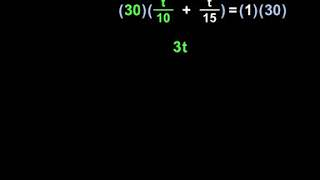By MathHelp.com

To solve a polynomial inequality, like the one shown here, our first step is to write the corresponding equation. In other words, we simply change the inequality sign to an equals sign, and we have x^2 – 3 = 9 – x. Next, we solve the equation. Since we have a squared term, we first set the equation equal to 0. So we move the 9 – x to the left side by subtracting 9 and adding x to both sides of the equation. This gives us x^2 + x – 12 = 0. Next, we factor the left side as the product of two binomials. Since the factors of negative 12 that add to positive 1 are positive 4 and negative 3, we have x + 4 times x – 3 = 0. So either x + 4 = 0 or x – 3 = 0, and solving each equation from here, we have x = -4, and x = 3. Now, it’s important to understand that the solutions to the equation, -4 and 3, represent what are called the “critical values” of the inequality, and we plot these critical values on a number line. However, notice that our original inequality uses a greater than sign, rather than greater than or equal to sign, so we use open dots on our critical values of -4 and positive 3. Remember that ‘greater than’ or ‘less than’ means open dot, and ‘greater than or equal to’ or ‘less than or equal to’ means closed dot. Now, we can see that our critical values have divided the number line into three separate intervals: less than -4, between -4 and 3, and greater than 3. And here’s the important part. Our next step is to test a value from each of the intervals by plugging the value back into the original inequality to see if it gives us a true statement. So let’s first test a value from the “less than -4” interval, such as -5. If we plug a -5 back in for both x’s in the original inequality, we have -5 squared – 3 greater than 9 minus a -5, which simplifies to 25 – 3 greater than 9 + 5, or 22 greater than 14. Since 22 greater than 14 is a true statement, this means that all values in the interval we’re testing are solutions to inequality, so we shade the interval. Next, we test a value from the “between -4 and 3” interval, such as 0. If we plug a 0 back in for both x’s in the original inequality, we have 0 squared – 3 greater than 9 – 0, which simplifies to 0 – 3 greater than 9, or -3 greater than 9. Since -3 greater than 9 is a false statement, this means that all values in the interval we’re testing are not solutions to inequality, so we don’t shade the interval. Next, we test a value from the “greater than 3” interval, such as 4. If we plug a 4 back in for both x’s in the original inequality, we have 4 squared – 3 greater than 9 – 4, which simplifies to 16 – 3 greater than 5, or 13 greater than 5. Since 13 greater than 5 is a true statement, this means that all values in the interval we’re testing are solutions to inequality, so we shade the interval. Finally, we write the answer that’s shown on our graph in set notation. The set of all x’s such that x is less than -4 or x is greater than 3.# Solving Compound Inequalities | MathHelp.com

##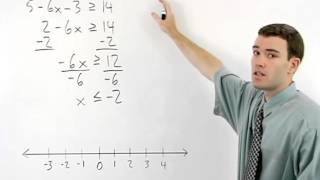By MathHelp.com# Solving Inequalities | MathHelp.com

##By MathHelp.com# Maths Problems - MathHelp.com - 1000+ Online Maths Lessons

##By MathHelp.com# TEXES Math Test Preparation - MathHelp.com

##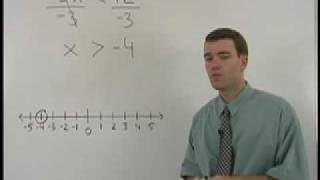By MathHelp.com# HESI Math Test Prep - MathHelp.com

##By MathHelp.com# Polynomial Inequalities | MathHelp.com

##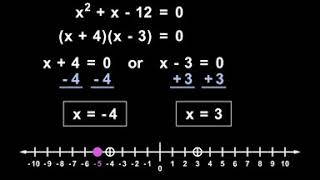By MathHelp.com# 08 - Solving Exponential Equations - Part 1 - Solve for the Exponent

##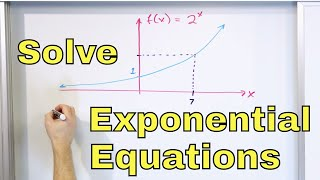By Math and Science

Quality Math And Science Videos that feature step-by-step example problems!# 06 - Review of Essential Trigonometry (Sin, Cos, Tangent - Trig Identities & Functions)

##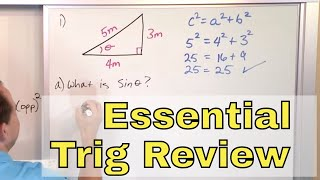By Math and Science

Quality Math And Science Videos that feature step-by-step example problems!